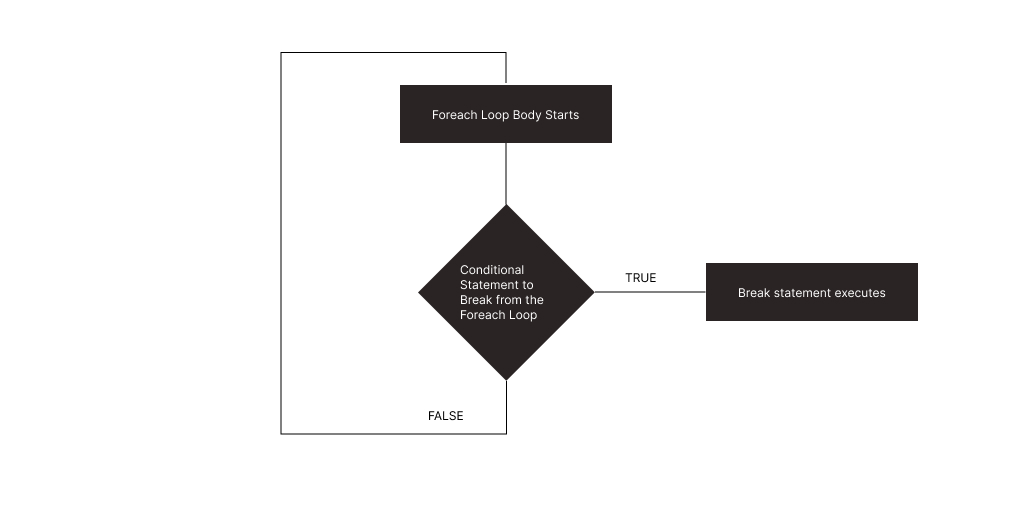# Break Out of the Foreach Loop in PHP

Olorunfemi Akinlua Jun 07, 2022

As developers, we use the `break` statement to break out of a loop and resume at the next statement following the loop. Often, a condition has to be set for such to happen, but it is not important.

You will often find breaks in `for` loops, `while` loops, `switch` statements, and even `foreach` loops. Therefore, to terminate any loop based on some condition, especially when the number of iterations is unknown, `break` is employed.

In this article, we will consider how to incorporate the `break` statement inside the `foreach` loop, the use cases, and the things not to forget.

## the `foreach` Loop in PHP

Before we get into how we can use `break` statements, let us have a simple refresher on `foreach` loops. Unlike the `for` loop, where we know in advance how many times we want to iterate, `foreach` loops iterate and go over an array via an array pointer and assign each array element to an array variable.

Say we are looking for a number, value, or string within an array but don’t know where the element is in the array and need it for another operation. We can use the `break` statement to leave the loop once we find the number, value, or string we need.

Example code:

``````<?php
\$colors = array("red", "green", "blue", "yellow");

foreach (\$colors as \$value) {
echo "\$value <br>";
}
?>
``````

Output:

``````red <br>green <br>blue <br>yellow <br>
``````

## Break Out of the `foreach` Loop Using the `break` Statement in PHP

The image below describes how the `break` statement works within a `foreach` loop. The `foreach` loop iterates over each array element and assigns it to the variable declared within the loop declaration.Within every iteration, the loop code block uses the current element the array pointer points at and tests for the conditional that will execute the `break` operation. If the conditional is true, the code breaks out of the loop, and if false, it continues to the next iteration.

Now, understanding the way the `break` statement works, let us consider a simple `foreach` example.

``````<?php
foreach (array('1','2','3') as \$first) {
echo "\$first ";
foreach (array('3','2','1') as \$second) {
echo "\$second ";
if (\$first == \$second) {
break;  // this will break both foreach loops
}
}
echo ". ";  // never reached!
}
echo "Loop Ended";
?>
``````

Output:

``````1 3 2 1 . 2 3 2 . 3 3 . Loop Ended
``````

We loop over every element in the code above and carry out the block code within the `foreach` loop using the current element.

We can use `break` statements in the `foreach` loop for any type of array, such as associative arrays. Here, once `\$x` reaches the middle array element, it stops the `foreach` loop.

``````<?php
\$age = array("Peter"=>"35", "Ben"=>"37", "Joe"=>"43");

foreach(\$age as \$x => \$val) {
echo "\$x = \$val<br>";
if (\$x == "Ben") {
break;
}
}
?>
``````

Output:

``````Peter = 35<br>Ben = 37<br>
``````

Remember, a conditional is useful for a successful `break` operation.

Olorunfemi is a lover of technology and computers. In addition, I write technology and coding content for developers and hobbyists. When not working, I learn to design, among other things.